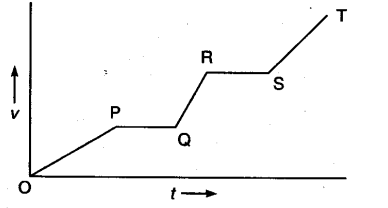# Give the interval in which magnitude of maximum acceleration is available

Give the interval in which magnitude of maximum acceleration is available.Slope of v-t graph gives acceleration so QR is the interval which gives maximum slope, QR is the interval which gives maximum acceleration.